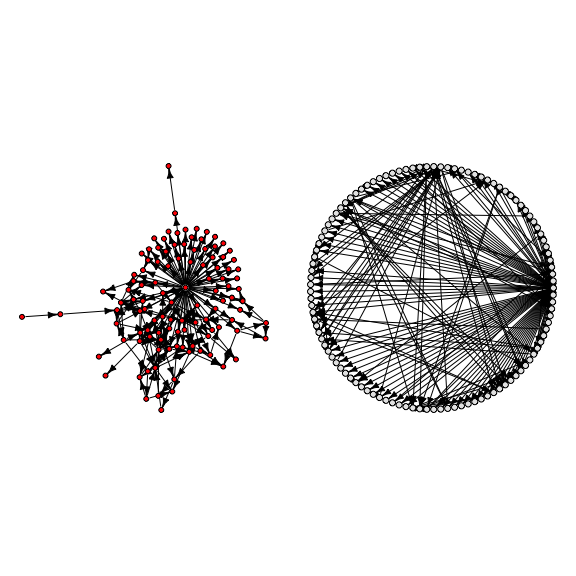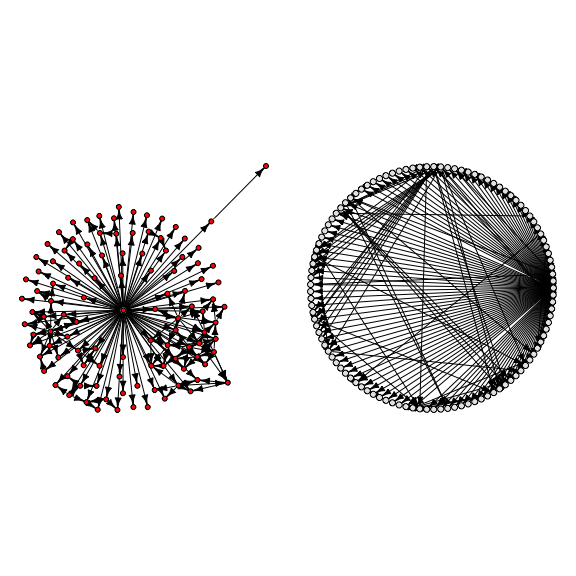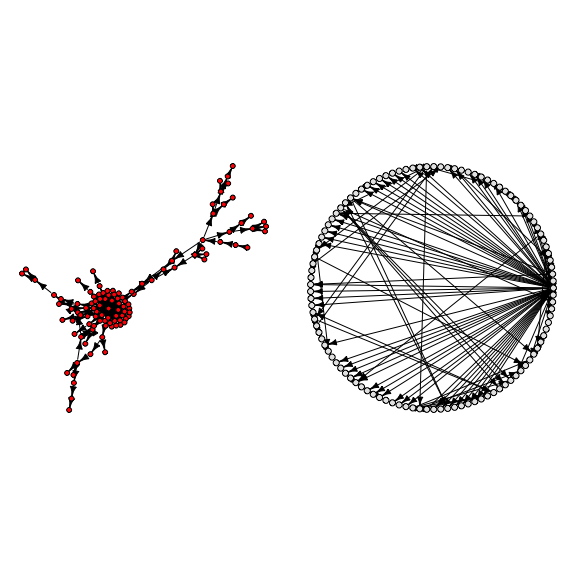# Introduction to the sparsebn package

#### 2020-09-10

sparsebn is an R package for learning sparse Bayesian networks and other graphical models from high-dimensional data via sparse regularization. It is designed to handle:

• Experimental data with interventions
• Mixed observational / experimental data
• High-dimensional data with p >> n
• Datasets with thousands of variables (tested up to p=8000)
• Continuous and discrete data

The main methods for learning graphical models are:

• estimate.dag for directed acyclic graphs (Bayesian networks).
• estimate.precision for undirected graphs (Markov random fields).
• estimate.covariance for covariance matrices.

Currently, estimation of precision and covariances matrices is limited to Gaussian data.

## tl;dr: Structure learning in five lines of code

The following example illustrates how to use sparsebn to learn a Bayesian network in just a few lines of code. An explanation of this code can be found in the next section.

library(sparsebn)
data(pathfinder)
data <- sparsebnData(pathfinder[["data"]], type = "continuous")
dags <- estimate.dag(data)
dags
## sparsebn Solution Path
##  109 nodes
##  1000 observations
##  16 estimates for lambda in [0.8338, 31.6228]
##  Number of edges per solution: 0-67-108-122-152-161-171-186-201-206-210-240-321-477-724-1069

## Example: Learning the pathfinder network

In this section, we will reconstruct the pathfinder network from the Bayesian network repository. The pathfinder network has 109 nodes and 195 edges.

In order to use the methods in the sparsebn package, we need to indicate what kind of data we are working with by wrapping the data into a sparsebnData object. First we load the data and then create a sparsebnData object:

data(pathfinder)
dat <- sparsebnData(pathfinder$data, type = "continuous", ivn = NULL) ## A list of interventions was not specified: Assuming data is purely observational. The sparsebnData object keeps track of what kind of data we are working with: Is it discrete or continuous, does it contain any interventions, and if it is discrete, what are the factor levels in the data? The argument type = "continuous" specifies that this data is continuous, and ivn = NULL indicate that there are no interventions. Now we can run the algorithm: dags <- estimate.dag(data = dat) dags ## sparsebn Solution Path ## 109 nodes ## 1000 observations ## 16 estimates for lambda in [0.8338, 31.6228] ## Number of edges per solution: 0-67-108-122-152-161-171-186-201-206-210-240-321-477-724-1069 Instead of automatically generating a grid of regularization parameters, we can also generate one manually (see ?generate.lambdas). nn <- num.samples(dat) # number of samples in the dataset / equivalent to nrow(dat$data)
lambdas <- generate.lambdas(sqrt(nn), 0.05, lambdas.length = 50, scale = "linear")
dags <- estimate.dag(data = dat,
lambdas = lambdas,
verbose = FALSE)
dags
## sparsebn Solution Path
##  109 nodes
##  1000 observations
##  50 estimates for lambda in [1.5811, 31.6228]
##  Number of edges per solution: 0-17-22-29-34-37-39-50-58-59-63-63-64-64-65-78-103-108-108-108-108-108-108-108-108-108-108-108-108-108-113-115-119-121-121-121-124-130-135-137-139-144-153-166-180-189-206-219-249-370

Note that the output is a solution path (stored internally as a sparsebnPath object), instead of a single estimate. In order to select a particular DAG, we need to do model selection. For example, we can visualize the solution with 195 edges:

solution <- select(dags, edges = 195)
par(mfrow = c(1,2), oma = rep(0,4))
plotDAG(solution)
plot(solution,
layout = igraph::layout_(to_igraph(solution$edges), igraph::in_circle()), vertex.label = NA, vertex.size = 5, vertex.label.color = gray(0), vertex.color = gray(0.9), edge.color = gray(0), edge.arrow.size = 0.45 )On the left, we use the plotDAG method, which uses some sensible defaults for plotting large graphs. On the right, we use the plot method (by default, imported from the igraph package) in order to use an organized circular layout and change some graphical parameters. The sparsebn package allows the user to use the igraph, network, or graph packages for visualization via the plot method. See ?setPlotPackage for more details. For comparison, let’s plot the original pathfinder graph; note that the plot on the right makes it easier to compare this to the previous plot. par(mfrow = c(1,2), oma = rep(0,4)) plotDAG(pathfinder$dag)
plot(pathfinder$dag, layout = igraph::layout_(to_igraph(pathfinder$dag), igraph::in_circle()),
vertex.label = NA,
vertex.size = 5,
vertex.label.color = gray(0),
vertex.color = gray(0.9),
edge.color = gray(0),
edge.arrow.size = 0.45
)Alternatively, we can automatically select a good solution using select.parameter:

select.idx <- select.parameter(dags, dat)
solution <- select(dags, index = select.idx) # same as dags[[select.idx]]

par(mfrow = c(1,2), oma = rep(0,4))
plotDAG(solution)
plot(solution,
layout = igraph::layout_(to_igraph(solution$edges), igraph::in_circle()), vertex.label = NA, vertex.size = 5, vertex.label.color = gray(0), vertex.color = gray(0.9), edge.color = gray(0), edge.arrow.size = 0.45 )The output of estimate.dag is a list of graphs, i.e. adjacency lists without edge weights. In order to estimate the edge weights, we use estimate.parameters: dags.fit <- estimate.parameters(dags, data = dat) The output is a list of weights, one for each value of $$\lambda$$. The weights are given in terms of $$(B,\Omega)$$, corresponding to the list list(coefs, vars). For example, we can see how the weight of the first node on the second changes as we decrease $$\lambda$$: unlist(lapply(dags.fit, function(x) x$coefs[1,2]))
##   0.000000 0.000000 0.000000 0.000000 0.000000 0.000000 0.000000 0.000000
##   0.000000 0.000000 0.000000 0.000000 0.000000 0.000000 0.000000 1.040467
##  1.040467 1.040467 1.040467 1.040467 1.040467 1.040467 1.040467 1.040467
##  1.040467 1.040467 1.040467 1.040467 1.040467 1.040467 1.040467 1.040467
##  1.040467 1.040467 1.040467 1.040467 1.040467 1.040467 1.040467 1.040467
##  1.040467 1.040467 1.040467 1.040467 1.040467 1.040467 1.040467 1.040467
##  1.040467 1.040467

## Example: Learning a Markov Chain

In this example, we illustrate how to learn a simple Markov chain on three nodes. We will also discuss how to use sparsebn to do covariance estimation. Suppose that the data is generated by the following graphical model:

$X_1\to X_2\to X_3.$

Assume unit influences between variables, i.e. $$X_j\sim\mathcal{N}(0,1)$$ and $$X_{j} = X_{j-1} + \varepsilon_j$$ with $$\varepsilon_j\sim\mathcal{N}(0,1)$$ for $$j>1$$. If $$X=(X_1,X_2,X_3)$$ then $$X=B^TX+\varepsilon\sim\mathcal{N}(0,\Sigma)$$, where we use the following parameters:

$B = \begin{pmatrix} 0 & 1 & 0 \\ 0 & 0 & 1 \\ 0 & 0 & 0 \end{pmatrix}, \quad \Omega = \begin{pmatrix} 1 & 0 & 0 \\ 0 & 1 & 0 \\ 0 & 0 & 1 \end{pmatrix}, \quad \Sigma = \begin{pmatrix} 3 & 2 & 1 \\ 2 & 2 & 1 \\ 1 & 1 & 1 \end{pmatrix}.$

To generate data from this model, first define the covariance matrix:

mean.vector <- rep(0, 3)
covariance.matrix <- rbind(c(3,2,1),
c(2,2,1),
c(1,1,1))

Then we can generate some data using the mvtnorm package:

gaussian.data <- mvtnorm::rmvnorm(n = 100, mean = mean.vector, sigma = covariance.matrix)
colnames(gaussian.data) <- c("X1", "X2", "X3")

Now we can use this data to estimate $$B$$:

dat <- sparsebnData(gaussian.data, type = "continuous")
## A list of interventions was not specified: Assuming data is purely observational.
dags <- estimate.dag(data = dat,
lambdas.length = 20,
edge.threshold = 10,
verbose = FALSE)
dags
## sparsebn Solution Path
##  3 nodes
##  100 observations
##  20 estimates for lambda in [0.1, 10]
##  Number of edges per solution: 0-0-2-2-2-2-2-2-2-2-2-2-2-2-2-2-2-2-2-2

As expected, the third estimate in our solution path gives the correct estimate:

dags[]
## CCDr estimate
## 100 observations
## lambda = 6.15848211066027
##
## DAG:
## [X1]
## [X2]  X1
## [X3]  X2
get.adjacency.matrix(dags[])
## 3 x 3 sparse Matrix of class "dgCMatrix"
##    X1 X2 X3
## X1  .  1  .
## X2  .  .  1
## X3  .  .  .

We can also use this data to directly estimate the covariance matrix $$\Sigma$$:

cov.out <- estimate.covariance(data = dat)

Compared with the output of estimate.dag, which is a more complicated sparsebnPath object, the output of estimate.covariance (and also estimate.precision) is simply a list of matrices:

class(cov.out)
##  "list"

Let’s take a look at the third estimate in the solution path (corresponding to the correct estimate of $$B$$ from before):

cov.out[]
## 3 x 3 sparse Matrix of class "dgCMatrix"
##
## [1,] 2.9379984 1.7640077 0.8451531
## [2,] 1.7640077 1.7477156 0.8373474
## [3,] 0.8451531 0.8373474 0.7831115

If we increase our sample size to $$n=1000$$, the estimate gets closer to the truth:

gaussian.data <- mvtnorm::rmvnorm(n = 1000, mean = mean.vector, sigma = covariance.matrix)
dat <- sparsebnData(gaussian.data, type = "continuous")
cov.out <- estimate.covariance(data = dat)
cov.out[]
## 3 x 3 sparse Matrix of class "dgCMatrix"
##
## [1,] 3.068878 2.073664 1.067335
## [2,] 2.073664 2.095356 1.078500
## [3,] 1.067335 1.078500 1.078698

## Appendix: Simulating data from a linear Gaussian SEM

Both datasets above have been simulated from a linear Gaussian SEM. In this section, we illustrate how to do this from scratch.

We first need a DAG; for this example we will use the pathfinder network. First, load this DAG:

data(pathfinder)
B <- as.matrix(get.adjacency.matrix(pathfinder$dag)) # pathfinder network as an adjacency matrix If $$X=B^TX+\varepsilon\sim\mathcal{N}(0,\Sigma)$$ and $$\varepsilon\sim\mathcal{N}(0,\Omega)$$, then one can show that: $\Sigma = (I-B)^{-T}\Omega(I-B)^{-1}.$ Assuming unit influences (i.e. $$\beta_{ij}=1$$ if $$\beta_{ij}\ne 0$$) and unit variances (i.e. $$\omega_j^2=1$$ for all $$j$$), we can then compute $$\Sigma$$ by using the above equation: id <- diag(rep(1, num.nodes(pathfinder$dag)))   # 109x109 identity matrix
Omega <- id                                     # conditional variances
Sigma <- solve(t(id - B)) %*% Omega %*% solve(id - B)

Finally, we can use the mvtnorm package to generate random multivariate Gaussian data:

set.seed(123)
nsamples <- 1000
gaussian.data <- mvtnorm::rmvnorm(nsamples, sigma = Sigma)

Instead of setting $$\beta_{ij}=1$$, we can also use random edge weights:

B[B!=0] <- runif(n = num.edges(pathfinder\$dag), min = 0.5, max = 2)
Sigma <- solve(t(id - B)) %*% Omega %*% solve(id - B)
gaussian.data <- mvtnorm::rmvnorm(nsamples, sigma = Sigma)